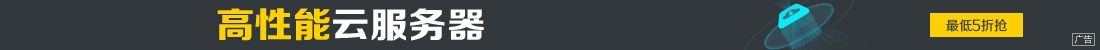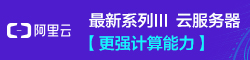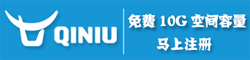# python 中if else 语句的作用及示例代码

••if-else语句语法结构

if 判断条件：

else：

```username= input("请输入用户名：")
print("登录成功！")
else:
print("用户名或者密码错误！")
# lower()--把字符串转为小写 upper()--把字符串转为大写
# strip()--去除字符串前后的空格```

C:\python\python.exeC:/python/demo/file2.py

Process finished with exit code 0

1）如果所有科目都及格了，提示：恭喜你，你所有科目都通过考试了

2）否则提醒：很遗憾，你没有通过考试，需要补考（没有及格的名称）

```chinese= int(input("请输入语文成绩："))
maths = int(input("请输入数学成绩："))
english = int(input("请输入英语成绩："))
get_course = ""
if chinese>= 60 and maths >= 60 and english>= 60:
print("恭喜你，所有科目都通过考试！")
else:
if chinese <= 60:
get_course += "语文、"
if maths <= 60:
get_course += "数学、"
if english <= 60:
get_course += "英语、"
print("很遗憾，你没有通过考试，补考科目为：" + get_course)```

C:\python\python.exeC:/python/demo/file2.py

Process finished with exit code 0

1）如果有一门是100分

2）如果有两门大于90分

3）如果三门大于80分

```chinese= int(input("请输入语文成绩："))
maths = int(input("请输入数学成绩："))
englist = int(input("请输入英语成绩："))
get_course = ""
if (chinese == 100 or maths == 100 or englist == 100):
if(chinese == 100):get_course += "语文、"
if(maths == 100):get_course += "数学、"
if(englist == 100):get_course += "英语、"
print("你的%s得了100分，奖励一朵小红花❀！" % get_course)
else:
if(chinese >= 90 and maths >=90) or (chinese >= 90 and englist>= 90) or(maths >= 90and englist >= 90):
if(chinese >= 100):get_course += "语文、"
if(maths >= 90):get_course += "数学、"
if(englist >= 90):get_course += "英语、"
print("你的%s大于90分，奖励一朵小红花❀！" % get_course)
else:
if(chinese >= 80 and maths >=80 and englist >= 80):
print("你的三个科目语文、数学、英语都大于80分，奖励一朵小红花❀")```

C:\python\python.exeC:/python/demo/file2.py